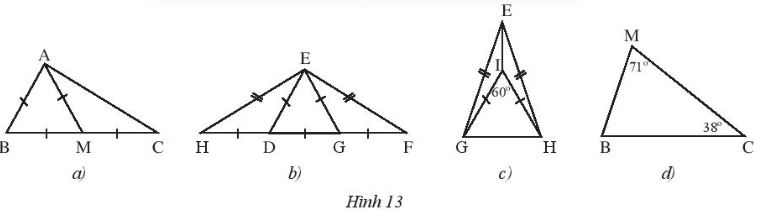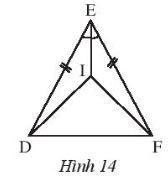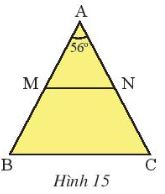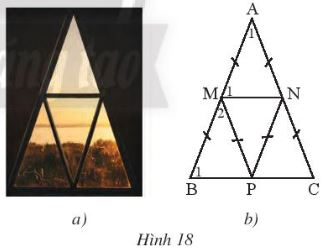## Solution of Exercise 3: Isosceles triangle (C8 Math 7 Horizon) – Math Book

Solution of Exercise 3: Isosceles triangle (C8 Math 7 Horizon)
===========

### Solve problem 1 page 62 Math textbook 7 Creative horizon volume 2 – CTST

Find the isosceles and equilateral triangles in each of the following figures (Figure 13). Explain.Detailed instructions for solving Lesson 1

Solution method

Based on the sides and the measure of the base angles of each triangle

Detailed explanation

a) Triangle ABM is an equilateral triangle because it has 3 equal sides

Triangle AMC is isosceles at M since AM = MC

b) Triangle EDG is an equilateral triangle because it has 3 equal sides

Triangle EHF is isosceles at E since EH = EF

Triangle EDH is isosceles at D since DH = DE

c) The triangle EGF is isosceles at G since GE = GF

Triangle IHG is equilateral because it is an isosceles triangle with 1 angle = 60°

Triangle EHG is isosceles at E since EG = EH

d) triangle MBC is not isosceles and irregular because the 3 angles have different measures

–>

— *****

### Solve problem 2, page 62, Math 7 Textbook, Creative horizon, volume 2 – CTST

Given Figure 14, know ED = EF and EI is the bisector of \(\widehat {DEF}\)Prove that:

a) \(\Delta EID = \Delta EIF\)

b) Isosceles triangle DIF

Detailed instructions for solving Lesson 2

Solution method

– We use the property cgc to prove statement a

– From sentence a, we infer ID = FI and prove that triangle DIF is isosceles

Detailed explanation

a) Consider triangle EID and triangle EIF with :

General IE

ED = EF

\(\widehat {IED} = \widehat {IEF}\)( EI is the bisector of \(\widehat {DEF}\))

\( \Rightarrow \Delta EID = \Delta EIF(c – g – c)\)

b) Since \(\Delta EID = \Delta EIF\) so ID = IF ( 2 corresponding edges )

Hence triangle DIF is isosceles at I (according to the definition of an isosceles triangle).

–>

— *****

### Solution 3 page 63 Math textbook 7 Creative horizon volume 2 – CTST

Let ABC be an isosceles triangle at A with \(\widehat A = {56^o}\)(Figure 15)

a) Calculate\(\widehat B\), \(\widehat C\)

b) Let M, N be the midpoints of AB and AC respectively. Prove that triangle AMN is isosceles.

c) Prove that MN // BCDetailed instructions for solving Lesson 3

Solution method

a) Use the summation theorem of 3 angles in a triangle and property of the 2 base angles of an isosceles triangle

b) Prove AM = AN

c) Use isotopic angle property

Detailed explanation

a) According to the problem, we have an isosceles triangle ABC at A and \(\widehat A = {56^o}\)

Where \( \Rightarrow \widehat A + \widehat B + \widehat C = {180^o}\)

\( \Rightarrow \widehat B = \widehat C = ({180^o} – {56^o}):2 = {62^o}\)

b) Since triangle ABC is isosceles at A, AB = AC (definition of isosceles triangle)

Where M, N are midpoints of AB, AC

So AM = AN

Consider triangle AMN with AM = AN, so AMN is an isosceles triangle at A

\( \Rightarrow \widehat M = \widehat N = ({180^o} – {56^o}):2 = {62^o}\)

c) Because \(\widehat {AMN}=\widehat {ABC}\) (same as 62°)

Since they are in isotopic positions, MN⫽BC

–>

— *****

### Solution 4 page 63 Math textbook 7 Creative horizon volume 2 – CTST

Let ABC be an isosceles triangle at A (Figure 16). The bisector of angle B intersects AC at F, the bisector of angle C meets AB at E.

a) Prove that \(\widehat {ABF} = \widehat {ACE}\)

b) Prove that triangle AEF is isosceles

c) Let I be the intersection of BF and CE. Prove that triangle IBC and triangle IEF are isosceles triangles

Detailed instructions for solving Lesson 4

Solution method

a) Use properties of isosceles triangle and bisector

b) From sentence a infer AE = AF

c) Triangle IEF proves isosceles by proving that the two sides are congruent

Prove that IBC is isosceles because the base angles are congruent

Detailed explanation

a) Since triangle ABC is isosceles at A

\( \Rightarrow \widehat B = \widehat C \Rightarrow \dfrac{1}{2}\widehat B = \dfrac{1}{2}\widehat C \Rightarrow \widehat {ABF} = \widehat {ACE}\ )

b) Consider \(\Delta ECA\) and \(\Delta FBA\) have:

\(\widehat{A}\) general

AB = AC

\(\widehat {ABF} = \widehat {ACE}\)

\( \Rightarrow \)\(\Delta ECA\)= \(\Delta FBA\)( g – c – g )

\( \Rightarrow AE = AF and EC = BF\) (2 sides respectively)

\( \Rightarrow \Delta AEF\) weighed at A

c) Consider triangle IBC with :

\(\widehat B = \widehat C \Rightarrow \dfrac{1}{2}\widehat B = \dfrac{1}{2}\widehat C \Rightarrow \widehat {ICB} = \widehat {IBC}\)

Therefore, triangle IBC is isosceles at I (the two base angles are congruent).

\( \Rightarrow IB = IC\)(corresponding edge )

Because EC = BF (sentence b) and IB = IC

\( \Rightarrow \) EC – IC = BF – BI

\( \Rightarrow \) EI = FI

\( \Rightarrow \Delta IEF\) weighed at I

–>

— *****

### Solving problems 5 pages 63 Math textbook 7 Creative horizon volume 2 – CTST

The body of a clothes hanger has the shape of an isosceles triangle (Figure 17a) as shown in Figure 17b. Let AB = 20 cm; BC = 28 cm and \(\widehat B\)= 35°. Find the measures of the remaining angles and perimeter of triangle ABC.Detailed instructions for solving Lesson 5

Solution method

Apply isosceles triangle property to find remaining angles and sides

Detailed explanation

Since triangle ABC is isosceles at A

\( \Rightarrow \) AB = AC ( isosceles triangle theorem ) = 20 cm

\( \Rightarrow \) Perimeter of triangle ABC = AB + AC + BC = 20 + 20 + 28 = 68 cm

Since ABC is an isosceles triangle at A \( \Rightarrow \widehat B = \widehat C\) ( 2 base angles ) = 35°

According to the theorem, sum of 3 angles in a triangle = 180°

\( \Rightarrow \widehat A = {180^o} – \widehat B – \widehat C = {180^o} – {35^o} – {35^o} = {110^o}\)

–>

— *****

### Solve problem 6 page 63 Math textbook 7 Creative horizon volume 2 – CTST

A triangular window frame of the design shown in Figure 18a is redrawn as shown in Figure 18b.

a) Indicates \(\widehat {{A_1}}\)\( = {42^o}\). Calculate the measure of \(\widehat {{M_1}}\),\(\widehat {{B_1}}\),\(\widehat {{M_2}}\).

b) Prove MN // BC, MP // AC.

c) Prove that the four isosceles triangles AMN, MBP, PMN, and NPC are congruent.Detailed instructions for solving Lesson 6

Solution method

Using properties of isosceles triangles

Detailed explanation

a) We see that triangle AMN is isosceles at A because AM = AN

\( \Rightarrow \widehat {{M_1}} = ({180^o} – \widehat {{A_1}}):2 = ({180^o} – {42^o}):2 = {69^o }\)

We see triangle PMN = triangle AMN ( ccc )

\( \Rightarrow \widehat {{M_1}} = \widehat {PMN} = {69^o}\) (corresponding angle )

Which \( \Rightarrow \widehat {{M_1}} + \widehat {{M_2}} + \widehat {PMN} = {180^o}\)( complementary angles )

\( \Rightarrow \widehat {{M_2}} = {180^o} – {69^o} – {69^o} = {42^o}\)

And triangle MPB is isosceles at M because MB = MP, so

\( \Rightarrow \widehat {{B_1}} = \widehat {MPB}\)

Apply the sum of the three angles theorem in a triangle

\( \Rightarrow \widehat {{B_1}} = ({180^o} – {42^o}):2 = {69^o}\)

b) We see that \(\widehat {{B_1}}\) and \(\widehat {{M_1}}\) are isotopic and equal, so

\( \Rightarrow \)MN⫽BC

Since triangle PMN = triangle AMN, we have

\( \Rightarrow \widehat {{M_1}} = \widehat {ANM} = \widehat {PMN} = \widehat {MNP}\)( due to 2 isosceles and congruent triangles )

Where \(\widehat {MNA}\) and \(\widehat {PMN}\) are staggered in

\( \Rightarrow \)MP⫽AC

c) We have \(\Delta AMN = \Delta PMN = \Delta MBP(c – g – c)\)(1)

Because MP⫽AC (proven above)

\( \Rightarrow \widehat {MPN} = \widehat {PNC}\) ( 2 staggered angles in ) =\({42^o}\)

\( \Rightarrow \Delta MPN = \Delta NCP(c – g – c)\)(2)

From (1) and (2) \( \Rightarrow \) 4 isosceles triangles AMN, MBP, PMN, NCP are equal

–>

— *****